# Relative homological algebra

(diff) ← Older revision | Latest revision (diff) | Newer revision → (diff)

A homological algebra associated with a pair of Abelian categories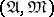and a fixed functor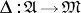(cf. Abelian category). The functoris taken to be additive, exact and faithful. A short exact sequence of objects of,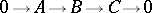is said to be admissible if the exact sequence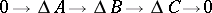splits in(cf. Split sequence). By means of the class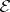of admissible exact sequences, the class of-projective (respectively,-injective) objects is defined as the class of those objects(respectively,) for which the functor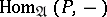(respectively,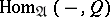) is exact on the admissible short exact sequences.

Any projective objectofis-projective, although this does not mean that inthere are enough relative projective objects (i.e. that for any objectfrom, an admissible epimorphism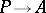of a certain-projective object ofexists). Ifcontains enough-projective or-injective objects, then the usual constructions of homological algebra make it possible to construct derived functors in this category, which are called relative derived functors.

Examples. Letbe the category of-modules over an associative ringwith a unit, letbe the category of Abelian groups and letbe the functor which "forgets" the module structure. In this case all exact sequences are admissible, and as a result the "absolute" (i.e. usual) homological algebra is obtained.

Ifis a group, then every-module is, in particular, an Abelian group. Ifis an algebra over a commutative ring, then every-module is a-module. Ifandare rings and, then every-module is an-module. In all these cases there is a functor from one Abelian category into the other defining the relative derived functors.

How to Cite This Entry:
Relative homological algebra. Encyclopedia of Mathematics. URL: http://encyclopediaofmath.org/index.php?title=Relative_homological_algebra&oldid=15425
This article was adapted from an original article by V.E. GovorovA.V. Mikhalev (originator), which appeared in Encyclopedia of Mathematics - ISBN 1402006098. See original article